# Kurtosis

In probability theory and statistics, kurtosis (from Greek: κυρτός, kyrtos or kurtos, meaning "curved, arching") is a measure of the "tailedness" of the probability distribution of a real-valued random variable. In a similar way to the concept of skewness, kurtosis is a descriptor of the shape of a probability distribution and, just as for skewness, there are different ways of quantifying it for a theoretical distribution and corresponding ways of estimating it from a sample from a population. Depending on the particular measure of kurtosis that is used, there are various interpretations of kurtosis, and of how particular measures should be interpreted.

The standard measure of kurtosis, originating with Karl Pearson, is based on a scaled version of the fourth moment of the data or population. This number measures heavy tails, and not peakedness; hence, the historical "peakedness" definition is wrong. For this measure, higher kurtosis means more of the variance is the result of infrequent extreme deviations, as opposed to frequent modestly sized deviations.

The kurtosis of any univariate normal distribution is 3. It is common to compare the kurtosis of a distribution to this value. Distributions with kurtosis less than 3 are said to be platykurtic, although this does not imply the distribution is "flat-topped" as sometimes reported. Rather, it means the distribution produces fewer and less extreme outliers than does the normal distribution. An example of a platykurtic distribution is the uniform distribution, which does not produce outliers. Distributions with kurtosis greater than 3 are said to be leptokurtic. An example of a leptokurtic distribution is the Laplace distribution, which has tails that asymptotically approach zero more slowly than a Gaussian, and therefore produces more outliers than the normal distribution. It is also common practice to use an adjusted version of Pearson's kurtosis, the excess kurtosis, which is the kurtosis minus 3, to provide the comparison to the normal distribution. Some authors use "kurtosis" by itself to refer to the excess kurtosis. For the reason of clarity and generality, however, this article follows the non-excess convention and explicitly indicates where excess kurtosis is meant.

Alternative measures of kurtosis are: the L-kurtosis, which is a scaled version of the fourth L-moment; measures based on four population or sample quantiles. These are analogous to the alternative measures of skewness that are not based on ordinary moments.

## Pearson moments

The kurtosis is the fourth standardized moment, defined aswhere μ4 is the fourth central moment and σ is the standard deviation. Several letters are used in the literature to denote the kurtosis. A very common choice is κ, which is fine as long as it is clear that it does not refer to a cumulant. Other choices include γ2, to be similar to the notation for skewness, although sometimes this is instead reserved for the excess kurtosis.

The kurtosis is bounded below by the squared skewness plus 1:where μ3 is the third central moment. The lower bound is realized by the Bernoulli distribution. There is no upper limit to the excess kurtosis of a general probability distribution, and it may be infinite.

A reason why some authors favor the excess kurtosis is that cumulants are extensive. Formulas related to the extensive property are more naturally expressed in terms of the excess kurtosis. For example, let X1, ..., Xn be independent random variables for which the fourth moment exists, and let Y be the random variable defined by the sum of the Xi. The excess kurtosis of Y iswhereis the standard deviation of. In particular if all of the Xi have the same variance, then this simplifies toThe reason not to subtract off 3 is that the bare fourth moment better generalizes to multivariate distributions, especially when independence is not assumed. The cokurtosis between pairs of variables is an order four tensor. For a bivariate normal distribution, the cokurtosis tensor has off-diagonal terms that are neither 0 nor 3 in general, so attempting to "correct" for an excess becomes confusing. It is true, however, that the joint cumulants of degree greater than two for any multivariate normal distribution are zero.

For two random variables, X and Y, not necessarily independent, the kurtosis of the sum, X + Y, isNote that the binomial coefficients appear in the above equation.

### Interpretation

The exact interpretation of the Pearson measure of kurtosis (or excess kurtosis) used to be disputed, but is now settled. As Westfall (2014) notes, "...its only unambiguous interpretation is in terms of tail extremity; i.e., either existing outliers (for the sample kurtosis) or propensity to produce outliers (for the kurtosis of a probability distribution)." The logic is simple: Kurtosis is the average (or expected value) of the standardized data raised to the fourth power. Any standardized values that are less than 1 (i.e., data within one standard deviation of the mean, where the "peak" would be), contribute virtually nothing to kurtosis, since raising a number that is less than 1 to the fourth power makes it close to zero. The only data values (observed or observable) that contribute to kurtosis in any meaningful way are those outside the region of the peak; i.e., the outliers. Therefore kurtosis measures outliers only; it measures nothing about the "peak."

Many incorrect interpretations of kurtosis that involve notions of peakedness have been given. One is that kurtosis measures both the "peakedness" of the distribution and the heaviness of its tail. Various other incorrect interpretations have been suggested, such as "lack of shoulders" (where the "shoulder" is defined vaguely as the area between the peak and the tail, or more specifically as the area about one standard deviation from the mean) or "bimodality". Balanda and MacGillivray assert that the standard definition of kurtosis "is a poor measure of the kurtosis, peakedness, or tail weight of a distribution" and instead propose to "define kurtosis vaguely as the location- and scale-free movement of probability mass from the shoulders of a distribution into its center and tails".

### Moors' interpretation

In 1986 Moors gave an interpretation of kurtosis. Letwhere X is a random variable, μ is the mean and σ is the standard deviation. Thenwhere κ is the kurtosis, var() is the variance and E() is the expectation operator. The kurtosis can now be seen to be a measure of the dispersion of Z2 around its expectation. Alternatively it can be seen to be a measure of the dispersion of Z around +1 and −1. κ attains its minimal value in a symmetric two-point distribution. In terms of the original variable X, the kurtosis is a measure of the dispersion of X around the two values μ ± σ.

High values of κ arise in two circumstances:

• where the probability mass is concentrated around the mean and the data-generating process produces occasional values far from the mean,
• where the probability mass is concentrated in the tails of the distribution.

## Excess kurtosis

The excess kurtosis is defined as kurtosis minus 3. There are 3 distinct regimes as described below.

### Mesokurtic

Distributions with zero excess kurtosis are called mesokurtic, or mesokurtotic. The most prominent example of a mesokurtic distribution is the normal distribution family, regardless of the values of its parameters. A distribution that has zero probability at countably many points on the real line and has a Gaussian shape everywhere else on the real line is likewise mesokurtic. A few other well-known distributions can be mesokurtic, depending on parameter values: for example, the binomial distribution is mesokurtic for.

### Leptokurtic

A distribution with positive excess kurtosis is called leptokurtic, or leptokurtotic. "Lepto-" means "slender". In terms of shape, a leptokurtic distribution has fatter tails. Examples of leptokurtic distributions include the Student's t-distribution, Rayleigh distribution, Laplace distribution, exponential distribution, Poisson distribution and the logistic distribution. Such distributions are sometimes termed super-Gaussian.

### Platykurtic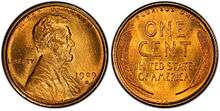The coin toss is the most platykurtic distribution

A distribution with negative excess kurtosis is called platykurtic, or platykurtotic. "Platy-" means "broad". In terms of shape, a platykurtic distribution has thinner tails. Examples of platykurtic distributions include the continuous or discrete uniform distributions, and the raised cosine distribution. The most platykurtic distribution of all is the Bernoulli distribution with p = ½ (for example the number of times one obtains "heads" when flipping a coin once, a coin toss), for which the excess kurtosis is −2. Such distributions are sometimes termed sub-Gaussian.

## Graphical examples

### The Pearson type VII family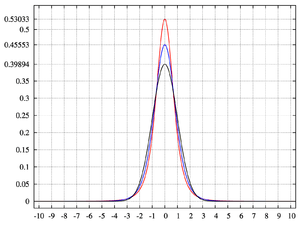pdf for the Pearson type VII distribution with excess kurtosis of infinity (red); 2 (blue); and 0 (black)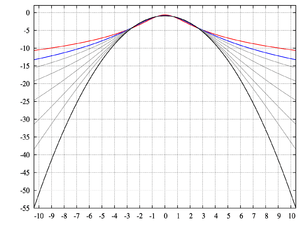log-pdf for the Pearson type VII distribution with excess kurtosis of infinity (red); 2 (blue); 1, 1/2, 1/4, 1/8, and 1/16 (gray); and 0 (black)

The effects of kurtosis are illustrated using a parametric family of distributions whose kurtosis can be adjusted while their lower-order moments and cumulants remain constant. Consider the Pearson type VII family, which is a special case of the Pearson type IV family restricted to symmetric densities. The probability density function is given bywhere a is a scale parameter and m is a shape parameter.

All densities in this family are symmetric. The kth moment exists provided m > (k + 1)/2. For the kurtosis to exist, we require m > 5/2. Then the mean and skewness exist and are both identically zero. Setting a2 = 2m  3 makes the variance equal to unity. Then the only free parameter is m, which controls the fourth moment (and cumulant) and hence the kurtosis. One can reparameterize with, whereis the excess kurtosis as defined above. This yields a one-parameter leptokurtic family with zero mean, unit variance, zero skewness, and arbitrary non-negative excess kurtosis. The reparameterized density isIn the limit asone obtains the densitywhich is shown as the red curve in the images on the right.

In the other direction asone obtains the standard normal density as the limiting distribution, shown as the black curve.

In the images on the right, the blue curve represents the densitywith excess kurtosis of 2. The top image shows that leptokurtic densities in this family have a higher peak than the mesokurtic normal density, although this conclusion is only valid for this select family of distributions. The comparatively fatter tails of the leptokurtic densities are illustrated in the second image, which plots the natural logarithm of the Pearson type VII densities: the black curve is the logarithm of the standard normal density, which is a parabola. One can see that the normal density allocates little probability mass to the regions far from the mean ("has thin tails"), compared with the blue curve of the leptokurtic Pearson type VII density with excess kurtosis of 2. Between the blue curve and the black are other Pearson type VII densities with γ2 = 1, 1/2, 1/4, 1/8, and 1/16. The red curve again shows the upper limit of the Pearson type VII family, with(which, strictly speaking, means that the fourth moment does not exist). The red curve decreases the slowest as one moves outward from the origin ("has fat tails").

## Kurtosis of well-known distributions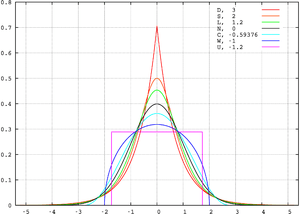Probability density functions for selected distributions with mean 0, variance 1 and different excess kurtosis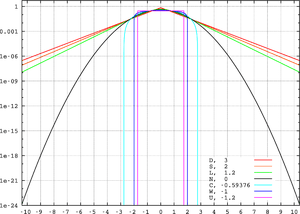Logarithms of probability density functions for selected distributions with mean 0, variance 1 and different excess kurtosis

Several well-known, unimodal and symmetric distributions from different parametric families are compared here. Each has a mean and skewness of zero. The parameters have been chosen to result in a variance equal to 1 in each case. The images on the right show curves for the following seven densities, on a linear scale and logarithmic scale:

Note that in these cases the platykurtic densities have bounded support, whereas the densities with positive or zero excess kurtosis are supported on the whole real line.

There exist platykurtic densities with infinite support,

and there exist leptokurtic densities with finite support.

• e.g., a distribution that is uniform between −3 and −0.3, between −0.3 and 0.3, and between 0.3 and 3, with the same density in the (−3, −0.3) and (0.3, 3) intervals, but with 20 times more density in the (−0.3, 0.3) interval

## Sample kurtosis

For a sample of n values the sample excess kurtosis iswhere m4 is the fourth sample moment about the mean, m2 is the second sample moment about the mean (that is, the sample variance), xi is the ith value, andis the sample mean.

This formula has the simpler representation,where thevalues are the standardized data values using the standard deviation defined using n rather than n  1 in the denominator.

For example, suppose the data values are 0, 3, 4, 1, 2, 3, 0, 2, 1, 3, 2, 0, 2, 2, 3, 2, 5, 2, 3, 999.

Then thevalues are −0.239, −0.225, −0.221, −0.234, −0.230, −0.225, −0.239, −0.230, −0.234, −0.225, −0.230, −0.239, −0.230, −0.230, −0.225, −0.230, −0.216, −0.230, −0.225, 4.359

and thevalues are 0.003, 0.003, 0.002, 0.003, 0.003, 0.003, 0.003, 0.003, 0.003, 0.003, 0.003, 0.003, 0.003, 0.003, 0.003, 0.003, 0.002, 0.003, 0.003, 360.976.

The average of these values is 18.05 and the excess kurtosis is thus 18.05  3 = 15.05. This example makes it clear that data near the "middle" or "peak" of the distribution do not contribute to the kurtosis statistic, hence kurtosis does not measure "peakedness." It is simply a measure of the outlier, 999 in this example.

## Sampling variance under normality

The variance of the sample kurtosis of a sample of size n from the normal distribution isStated differently, under the assumption that the underlying random variableis normally distributed, it can be shown that.

## Upper bound on kurtosis

An upper bound for the sample kurtosis of n (n > 2) real numbers is## Estimators of population kurtosis

Given a sub-set of samples from a population, the sample excess kurtosis above is a biased estimator of the population excess kurtosis. An alternative estimator of the population excess kurtosis is defined as follows:where k4 is the unique symmetric unbiased estimator of the fourth cumulant, k2 is the unbiased estimate of the second cumulant (identical to the unbiased estimate of the sample variance), m4 is the fourth sample moment about the mean, m2 is the second sample moment about the mean, xi is the ith value, andis the sample mean. Unfortunately,is itself generally biased. For the normal distribution it is unbiased.

## Applications

The sample kurtosis is a useful measure of whether there is a problem with outliers in a data set. Larger kurtosis indicates a more serious outlier problem, and may lead the researcher to choose alternative statistical methods.

D'Agostino's K-squared test is a goodness-of-fit normality test based on a combination of the sample skewness and sample kurtosis, as is the Jarque–Bera test for normality.

For non-normal samples, the variance of the sample variance depends on the kurtosis; for details, please see variance.

Pearson's definition of kurtosis is used as an indicator of intermittency in turbulence.

### kurtosis convergence

Applying band-pass filters to digital images, kurtosis values tend to be uniform, independent of the range of the filter. This behavior, termed kurtosis convergence, can be used to detect image splicing in forensic analysis.

## Other measures of kurtosis

A different measure of "kurtosis", that purports to measure the "peakedness" of a distribution, is provided by using L-moments instead of the ordinary moments.Wikimedia Commons has media related to Kurtosis.
1. Westfall, P.H. (2014), Kurtosis as Peakedness, 1905–2014. R.I.P., The American Statistician 68, 191–195.
2. Joanes, D. N.; Gill, C. A. (1998). "Comparing measures of sample skewness and kurtosis". Journal of the Royal Statistical Society (Series D): the Statistician. 47 (1): 183–189. doi:10.1111/1467-9884.00122.
3. Pearson, K. (1929). "Editorial note to 'Inequalities for moments of frequency functions and for various statistical constants'". Biometrika. 21 (1–4): 361–375. doi:10.1093/biomet/21.1-4.361.
4. Balanda, Kevin P.; MacGillivray, H.L. (1988). "Kurtosis: A Critical Review". The American Statistician. 42 (2): 111–119. doi:10.2307/2684482.
5. Darlington, Richard B (1970). "Is Kurtosis Really 'Peakedness'?". The American Statistician. 24 (2): 19–22. doi:10.1080/00031305.1970.10478885.
6. Balanda and MacGillivray, p. 114.
7. Moors JJA (1986b) The meaning of kurtosis: Darlington reexamined. The American Statistician 40 (4) 283–284
8. http://medical-dictionary.thefreedictionary.com/lepto-
9. http://www.yourdictionary.com/platy-prefix
10. The original paper presenting sub-Gaussians Kahane, J. P. (1960). "Propriétés locales des fonctions à séries de Fourier aléatoires" [Local properties of functions in terms of random Fourier series]. Stud. Math. 19 (1): 1–25. ISSN 0039-3223. See also Buldygin, V. V.; Kozachenko, Y. V. (1980). "Sub-Gaussian random variables". Ukrainian Mathematical Journal. 32 (6): 483–489. doi:10.1007/BF01087176.
11. Cramer, Duncan (1997). Fundamental Statistics for Social Research. Routledge. p. 89. ISBN 0-415-17204-7.
12. Kendall, M.G.; Stuart, A. (1969) The Advanced Theory of Statistics, Volume 1: Distribution Theory, 3rd Edition, Griffin. ISBN 0-85264-141-9
13. Sharma, R.; Bhandari, R. (11 Sep 2013). "Skewness, kurtosis and Newton's inequality". Rocky Mountain Journal of Mathematics. 45 (5): 1639–1643. arXiv:1309.2896.
14. Sandborn, V. A. (1959). "Measurements of Intermittency of Turbulent Motion in a Boundary Layer". Journal of Fluid Mechanics. 6 (2): 221–240. Bibcode:1959JFM.....6..221S. doi:10.1017/S0022112059000581.
15. Hosking, J.R.M. (1992). "Moments or L moments? An example comparing two measures of distributional shape". The American Statistician. 46 (3): 186–189. doi:10.2307/2685210. JSTOR 2685210.
16. Hosking, J.R.M. (2006). "On the characterization of distributions by their L-moments". Journal of Statistical Planning and Inference. 136: 193–198. doi:10.1016/j.jspi.2004.06.004.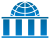Wikiversity has learning materials about Kurtosis